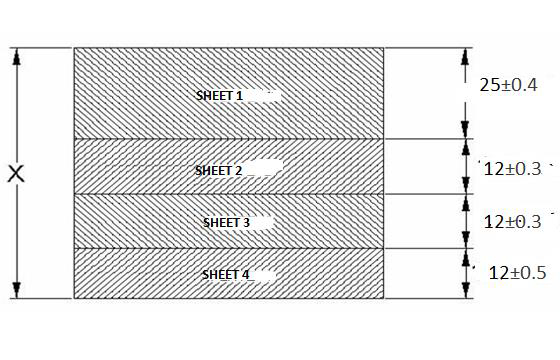# Tolerance Stack up

Data Structure AlgorithmsAnalysis of AlgorithmsAlgorithms

## What is Assembly Tolerance Stack up Analysis?

In short, assembly tolerance stacks up analysis is defined as the tolerance value of the whole assembly or a specific gap of the assembly when we are aware about the tolerance values of all its components.

Assembly tolerance chain stack up analysis can be accomplished in different ways. The simplest procedure is termed as the worst case method, which we discuss here.

## Discussion about Worst Case Method of Assembly Tolerance Stack Up

Let, we have an assembly of four thick sheets like below −The thickness and tolerance of the four sheets are displayed in the above figure. We have to calculate the dimension “X" and its tolerance value which is proceed as below −

We calculate the lower specification limit (LSL)size for each of the sheets like below −

For SHEET-1:
LSL= 25-0.4 = 24.6
For SHEET-2:
LSL= 12-0.3 = 11.7
For SHEET-3:
LSL= 12-0.3 = 11.7
For SHEET-4:
LSL= 12-0.5 = 11.5

We add the LSL thickness values of all the sheets, and will obtain the LSL thickness of the whole assembly as below

TL = 24.6 +11.7 + 11.7 + 11.5 = 59.5

We calculate the upper specification limit (USL)size for each of the sheets like below −

For SHEET-1:
USL= 25+0.4 = 25.4
For SHEET-2:
USL= 12+0.3 = 12.3
For SHEET-3:
USL= 12+0.3 = 12.3
For SHEET-4:
USL= 12+0.5 = 12.5

Add the USL thickness values of all the sheets, we obtain the USL of the whole assembly as below −

TU = 25.4 + 12.3 + 12.3 + 12.5 = 62.5

Tolerance of the whole assembly can be obtained as −

~+mn~ (TU – TL) / 2 = ~+mn~ (62.5-59.5)/2 = ~+mn~ 1.5

The nominal thickness dimensions of all the sheets are added to obtain the nominal thickness value of the whole assembly, like below −

TN = 25 + 12 + 12 + 12 = 61